ChaptersThis text is meant to accompany class discussions. It is not everything there is to know about uniform circular motion. It is meant as a  prep for class. More detailed notes and examples are given in the class notes, presentations, and demonstrations. See the links below.

Review

Energy

Kinetic energy is the energy of any moving body with mass. It's equation is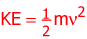m: v: KE: mass of a the body, [kg] velocity of the body, [m/s] kinetic energy, [J] ...All energies, n matter the adjective, are measured in Joules.

The only way to change the kinetic energy is to do work on the body.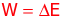Work done my a force opposing the body's direction of motion removes kinetic energy. Work done by a force that is applied in the same direction as the motion adds kinetic energy. The units of work are Joules. In terms of units, this is because work is equal to a change in "Joules." A Joule is a Newton•meter -when the force and displacement's directions are parallel to each other. Therefore:The energy of a SINGLE photon can be calculated from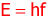h: f: Planck's constant 6.626 x 10-34 (m2•kg)/s Frequency [Hz] Hertz

The wave equation provides a way of converting between wavelength and frequency. The wave equation is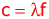λ: c: Wavelength [m] The wave speed, 3.00 x 108 m/s for electromagnetic waves in a vacuum

For a photon, its total energy is calculated from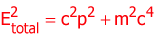p: c: particle's momentum [kgm/s] {Momentum is (mass)(velocity)} speed of light [m/s] ...it is a constant of 3.00 x 108 m/s in a vacuum.

If you were to annihilate a resting particle, (or any other body or piece of mass,) the energy released would be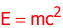Conservation Laws

For this course, there are three conservation laws that heve been emphasized.

• Conservation of energy
• Conservation of charge
• Conservation of mass

This means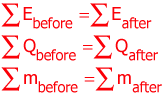Where the "before" and "after" refers to some event or condition.

Power

Power is define as how quickly energy is delivered or received.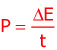P: ΔE: t: Power [W] Watts Energyfinal - Energyinitial [J] Joules time [s]

Momentum

Momentum is represented with the symbol "p."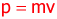Every object that is moving has momentum. Unlike energy, momentum is a vector. It has magnitude and direction. If the momentum changes, then an impulse was applied. This means a force was applied to the body. A change in momentum can be represented by a change in speed and/or a change in direction.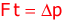The main "take-away" here is that when the momentum changes, a net force is present. In other words, when a body change speed or direction, a net force caused this to happen.

Electric Fields

Electric fields, (refereed to as e-fields,) provide a structure in space to apply a force to any body with a net charge. Electric fields can be controlled with multiple charges. Batteries are great sources of free charges. The typical setup for studying electric fields is shown below.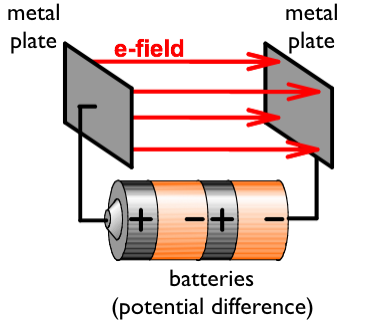The plate connected to the positive side of the bateries get a positive charge. The plate connected to the negative side of the batteries becomes negatively charged. The e-field is defined as the path a positive particle would travel. In this diagram above a positive particle would travel to the plate connected to the negative side of the battery.

The electric field is between the two plates is defined byE: d: ΔV: electric field [N/C] or [v/m] distance betweenthe plates [m] Potential difference acros the two surfaces [v]

As a particle travels from one plate the other the amount of work done is equal to qV.q: V: the particle's net charge, [C]: Coulombs is the potential difference of the power source, [v]: volts

This means that the energy lost or gained as a charged particle crosses the plates is equal to qV. Because the amounts of energy are so small they are measured in electronVolts, eV's.If a particle with a charge equal to a single elementaty charge, such as an electron or proton, travels across an electric field made by a battery, then the energy lost of gained equals the (# of elementary charges)x(the battery voltage) = eV's. Energy is lost of the opposite plate is the same sign as the particle and t is gained if the opposite plate is the opposite sign of the particle.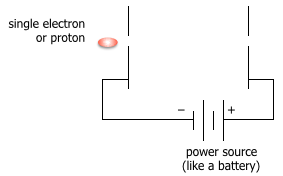For example: If the plates above are connected to a 12 volt battery, then an electron would gain 12 eV's of energy. A proton would lose 12 eV's of energy.

Example 2: If a particle with a charge equal to 3 elctrons travels between the plates while they are conencted to a 5 volt battery then the particle would gain (3)(5) = 15 eV's of energy.

Another important characteristic of an e-field is the fact that it can also guide charge particles. If we could create the e-field below, charges would travel around the corner.As it turns out, this is easily done with a conducting wire.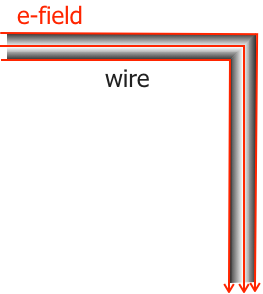This is because the electric field travels along the outside of the wire to move the charges in a current.

Current Electricity

Current is the flow of charge. It is defined as how quickly the charge flows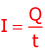I: Q: t: Current [A] Amps (properly refereed to as, "Amperes.") charge [C] Coulombs time [s]

To move charge from one end of wire to the other, a potential difference must exist between the two ends of the wire. A battery is a great source of a potential difference. Place the ends of the wire to opposite sides of the battery to get a potential difference on opposite ends of the wire equal to the battery's voltage. The potential difference is measured in volts. (As in the volts of the battery.)

Series Circuits:

A junction is where 3 or more wires meet. A series circuit is one where the components in series follow one another without any junctions between the components. Which elements are in series with each other?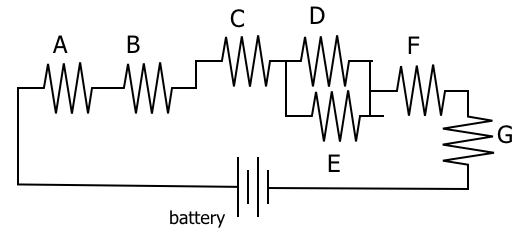The following are in series with each other, (F, G, battery, A, B,and C.)The current is the the same for all components in the same series. This is important because if you can calculate the current in any of these components, then that is the same current that is running through all of the other components in the same series. Click here for more informaiton and examples about series circuits.

Ohm's Law states that the voltage and current are propotional to each other across a simple resistor.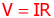V: I: R: Potential difference across an electrical component [V] (volts) Current [A] Amps (properly refereed to as, "Amperes.") Resistance [Ω] Ohms

Note: The charge of an electron is 1.6 x 10-19 C

by Tony Wayne ...(If you are a teacher, please feel free to use these resources in your teaching.)